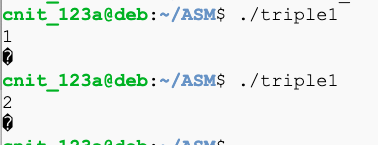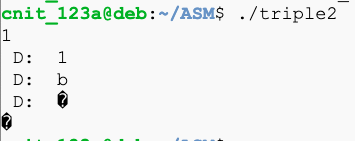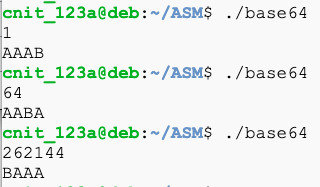# ASM 104: Bases & Printing (40 pts)

## What You Need

• A Google Cloud Debian 10 Linux Server

## Purpose

To practice using the oldest and simplest debugging technique: print statements.

Often you also need to use a real debugger like gdb, which is in a different challenge.

Create this "triple1.asm" file:
```section .text global _start _start: mov edx, len_buf_in mov ecx, buf_in mov ebx, 1 mov eax, 3 ; 3 = sys_read int 0x80 mov al, [buf_in] shl al, 1 ; double add al, al ; add one more mov [buf_out], al mov edx, len_buf_out mov ecx, buf_out mov ebx, 1 mov eax, 4 ; 4 = sys_write int 0x80 mov eax, 1 ; 1 = exit int 0x80 section .data buf_in db " ", 10, 13 len_buf_in equ \$ - buf_in buf_out db " ", 10, 13 len_buf_out equ \$ - buf_out ```
Compile and run the progam, as shown below.

The goal was to read numbers and triple them, but it fails, as shown below.Create this "triple2.asm" file:
```section .text global _start _start: mov edx, len_buf_in mov ecx, buf_in mov ebx, 1 mov eax, 3 ; 3 = sys_read int 0x80 mov al, [buf_in] call print_c ; diagnostic shl al, 1 ; double call print_c ; diagnostic add al, al ; add one more call print_c ; diagnostic mov [buf_out], al mov edx, len_buf_out mov ecx, buf_out mov ebx, 1 mov eax, 4 ; 4 = sys_write int 0x80 mov eax, 1 ; 1 = exit int 0x80 print_c: ; prints the character in al push eax mov [print_cs+5], al mov edx, len_print_cs mov ecx, print_cs mov ebx, 1 mov eax, 4 int 0x80 pop eax ret section .data buf_in db " ", 10, 13 len_buf_in equ \$ - buf_in buf_out db " ", 10, 13 len_buf_out equ \$ - buf_out print_cs db " D: ", 10, 13 len_print_cs equ \$ - print_cs ```
Compile and run the progam, as shown below.

Now it prints out the result after each step, as shown below. This makes it easier to find errors.## ASM 104.1: Hex (10 pts)

Create a program that accepts a decimal number and converts it to hex, as shown below.Convert this number to hex to obtain the flag:

```2880298733 ```

## ASM 104.2: Binary (10 pts)

Create a program that accepts a decimal number and converts it to binary, as shown below.Convert this number to binary to obtain the flag:

```2865539805 ```

## ASM 104.3: Base64 (10 pts)

Create a program that accepts a decimal number and converts it to Base64, as shown below.Convert this number to Base64 to obtain the flag:

```803075 ```

## ASM 104.4: Base58 (10 pts)

Create a program that accepts a decimal number and converts it to Base58Check, as shown below.Convert this number to Base58 to obtain the flag:

```807319263 ```

## Sources

System calls in the Linux kernel. Part 1.
X86 Assembly/Interfacing with Linux
Linux System Call Table (32-bit)
List of Linux/i386 system calls
Assembly Programming Tutorial

Posted 7-19-2020